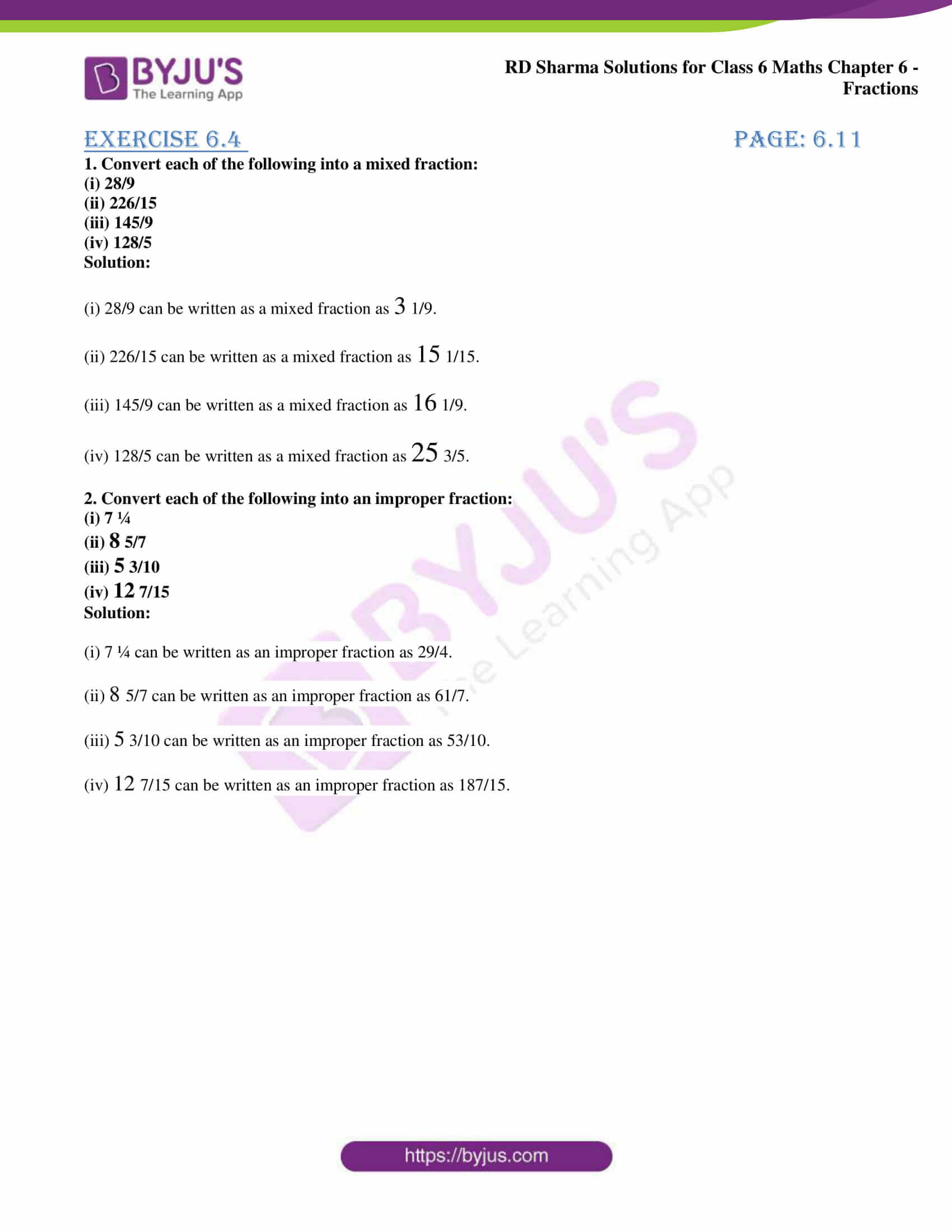# RD Sharma Solutions for Class 6 Maths Chapter 6: Fractions Exercise 6.4

RD Sharma Solutions for Class 6 are designed by highly knowledgeable experts at BYJU’S with the aim of helping students score well in the exam. The students can improve their logical thinking and problem solving abilities by solving exercise wise problems using PDF of solutions. Exercise 6.4 mainly provides knowledge about the various types of fractions and conversion of mixed fractions which are important from the exam point of view. The huge number of examples before each exercise helps students gain a clear idea about the concepts covered. PDF of RD Sharma Solutions for Class 6 Maths Chapter 6 Fractions Exercise 6.4 are present here.

## RD Sharma Solutions for Class 6 Maths Chapter 6: Fractions Exercise 6.4 Download PDF### Access answers to Maths RD Sharma Solutions for Class 6 Chapter 6: Fractions Exercise 6.4

1. Convert each of the following into a mixed fraction:

(i) 28/9

(ii) 226/15

(iii) 145/9

(iv) 128/5

Solution:

(i) 28/9 can be written as a mixed fraction as 3 1/9.

(ii) 226/15 can be written as a mixed fraction as 15 1/15.

(iii) 145/9 can be written as a mixed fraction as 16 1/9.

(iv) 128/5 can be written as a mixed fraction as 25 3/5.

2. Convert each of the following into an improper fraction:

(i) 7 ¼

(ii) 8 5/7

(iii) 5 3/10

(iv) 12 7/15

Solution:

(i) 7 ¼ can be written as an improper fraction as 29/4.

(ii) 8 5/7 can be written as an improper fraction as 61/7.

(iii) 5 3/10 can be written as an improper fraction as 53/10.

(iv) 12 7/15 can be written as an improper fraction as 187/15.